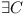'. '

RangeDependenciesNP

From the things described so far it seems that RangeDependencies may not be as bad, but still, there are severe NP-Complete problems associated with them. Following sections demonstrate the problems.

Module Repository with Ranges

Let A,B,C,... denote various modules and their APIs.

Let A1.0,A1.1,A1.7,A1.11,A2.1,A2.9,A3.0 denote versions of module A. It is not really important what format the versions use, the only condition is that there is a linear order among the versions. As such the rest of the terminology is using single variable (x or p) to denote the version.

Let$A_{x} \rightarrow B_{[u,v)}$ denote the fact that version x of module A depends on version range [u,v) of module B. E.g. any version of module B between u and less than v can be used to satisfy A's need. Let$A_{x} \rightarrow B_{[u,v]}$ denote closed interval dependency and allow its usage as well.

Let Repository R = (M,D) be any set of modules with their versions and their dependencies on other modules.

Let C be a Configuration in a repository R = (M,D), if$C \subseteq M$, where following is satisfied:

1. each dependency is satisfied with some version from dependency range:$\forall A_x \in C, \forall A_x \rightarrow B_{[u,v)} \in D \Rightarrow \exists w \in [u,v) \wedge B_{w} \in C$
2. only one version is enabled:$A_{x} \in C \wedge A_{y} \in C \Rightarrow x = y$

Module Range Dependency Problem

Let there be a repository R = (M,D) and a module$A \in M$. Does there exist a configuration C in the repository R, such that the module$A \in C$, e.g. the module can be enabled?

Conversion of 3SAT to Module Range Dependencies Problem

Let there be 3SAT formula with variables v1,...,vm as defined at in the original proof.

Let's create a repository of modules R. For each variable vi let's create two modules$M^i_{1.0}$ and$M^i_{1.1}$, and put them into repository R.

For each formula$(x_{i1} \vee x_{i2} \vee x_{i3})$ let's create a module Fi that will have three versions. Each of them will depend on one variable's module. In case the variable is used with negation, it will depend on version 1.0, otherwise on version 1.1. So for formula$v_a \vee \neg v_b \vee \neg v_c$

we will get:$F^i_{1.1} \rightarrow M^a_{[1.1,1.1]}$$F^i_{1.2} \rightarrow M^b_{[1.0,1.0]}$$F^i_{1.3} \rightarrow M^c_{[1.0,1.0]}$

All these modules and dependencies are added into repository R

Now we will create a module T1.0 that depends on all formulas:$T_{1.0} \rightarrow F^1_{[1.0,2.0)}$$T_{1.0} \rightarrow F^2_{[1.0,2.0)}$
...$T_{1.0} \rightarrow F^n_{[1.0,2.0)}$

and add this module as well as its dependencies into repository R.

Claim: There$\exists C$ (a configuration) of repository R and$T_{1.0} \in C$$\Longleftrightarrow$ there is a solution to the 3SAT formula.

The proof is step by step similar to the one given in LibraryReExportIsNPComplete, so it is not necessary to repeat it here.